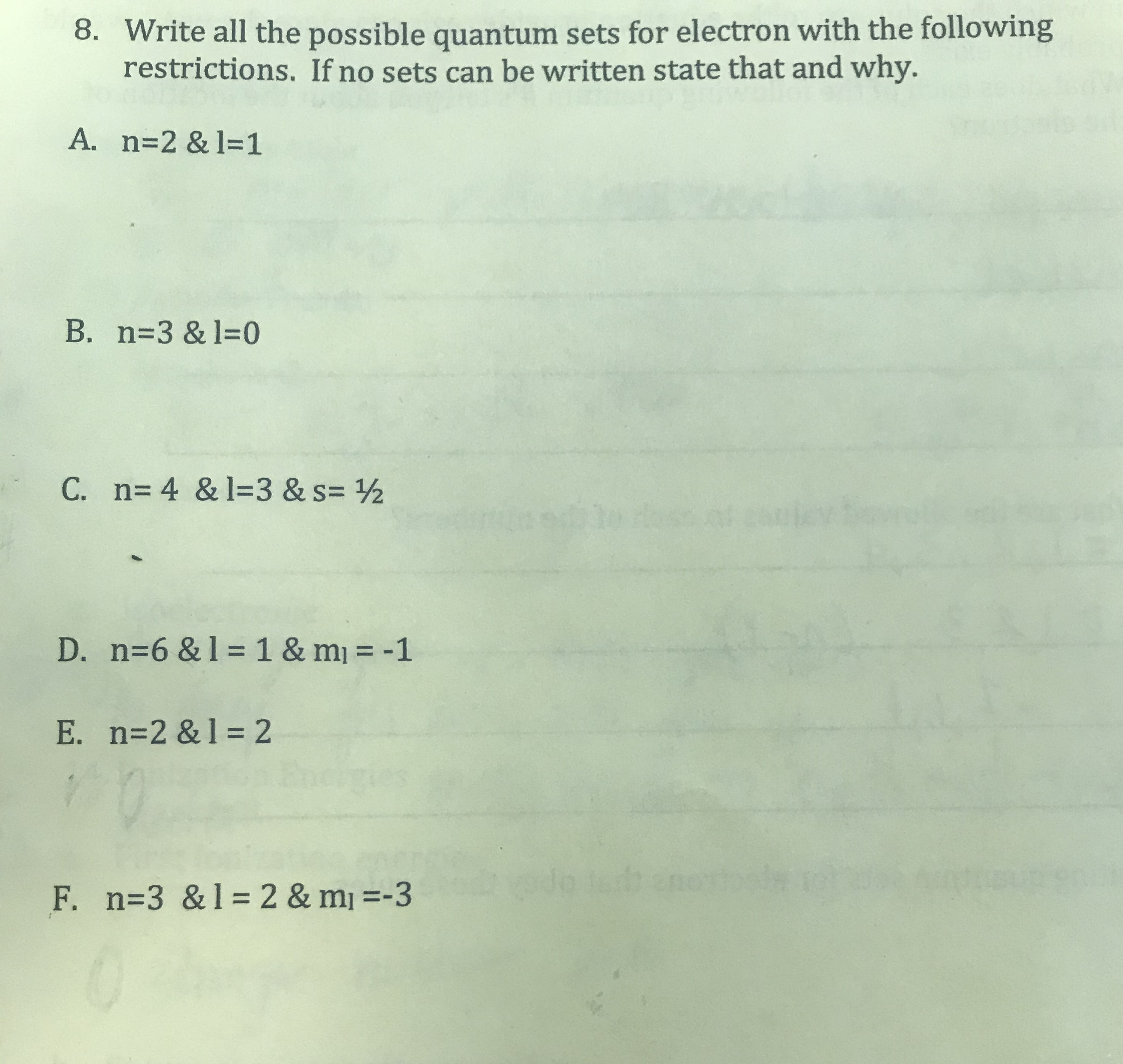# 8. Write all the possible quantum sets for electron with the followingrestrictions. If no sets can be written state that and why.A. n=2 & l=1B. n=3 & l=0C. n= 4 & 1=3 & s= ½D. n=6 & 1 =1 & m = -1%3DE. n=2 &1 = 2F. n=3 &1=2 & mj =-3

Question
1 viewshelp_outlineImage Transcriptionclose8. Write all the possible quantum sets for electron with the following restrictions. If no sets can be written state that and why. A. n=2 & l=1 B. n=3 & l=0 C. n= 4 & 1=3 & s= ½ D. n=6 & 1 =1 & m = -1 %3D E. n=2 &1 = 2 F. n=3 &1=2 & mj =-3 fullscreen
check_circle

Step 1

Principal quantum number:

n = 1 to ∞ (infinity).

n = 1,2,3,4,5…………..∞.

Azimuthal or angular momentum quantum number:

l = (n-1) values.

l = 0,1,2,3,      up to (n-1)

magnetic quantum number:

ml = -l to +l

spin quantum number:

ms = -1/2 or +1/2.Step 2- A. n = 2, l = 1

Principal quantum number:

n = 1 to ∞ (infinity).

n = 1,2,3,4,5…………..∞.

Azimuthal or angular momentum quantum number:

l = (n-1) values.

l =upto  (2 -1) values= 0, 1

Therefore, given set of quantum numbers(n = 2, l = 1) are possible.

Possible quantum numbers are:

 Shell n Subshell l Orientation ml Spin ms 2 1 1, 0, +1 -1/2 or +1/2.

Step 3: B. n = 3, l = 0.

Principal quantum number:

n = 1 to ∞ (infinity).

n = 1,2,3,4,5…………..∞.

Azimuthal or angular momentum quantum number:

l = (n-1) values.

l =  upto (3 -1) values

l = 0, 1, 2.

Therefore, given set of quantum numbers(n = 3, l = 0.) are possible.

Possible quantum numbers are:

 Shell n Subshell l Orientation ml Spin ms 3 0 0 -1/2 or +1/2.

...

### Want to see the full answer?

See Solution

#### Want to see this answer and more?

Solutions are written by subject experts who are available 24/7. Questions are typically answered within 1 hour.*

See Solution
*Response times may vary by subject and question.
Tagged in